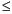Functions and CALL Routines

# PROBBNRM Function

Returns a probability from a bivariate normal distribution.
 Category: Probability

## Syntax

 PROBBNRM(x, y, r)

### Arguments

x

specifies a numeric constant, variable, or expression.

y

specifies a numeric constant, variable, or expression.

r

is a numeric correlation coefficient.

 Range: -1r1

The PROBBNRM function returns the probability that an observation (X, Y) from a standardized bivariate normal distribution with mean 0, variance 1, and a correlation coefficient r, is less than or equal to (x, y). That is, it returns the probability that Xx and Yy. The following equation describes the PROBBNRM function, where u and v represent the random variables x and y, respectively:SAS Statements Result
```p=probbnrm(.4, -.3, .2);
put p;```
```
0.2783183345```

 Functions:Previous Page | Next Page | Top of Page